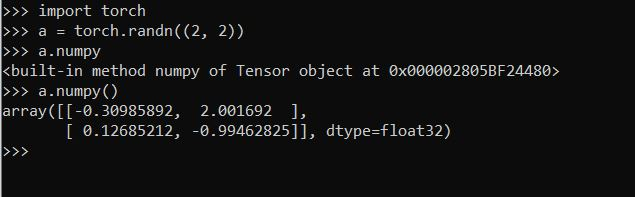# How to extract values from type built-in method numpy of Tensor object

I am running this following code to do prediction for my model. After I am trying plot a graph of practiced values - unfortunately the output of my variable Y_pred is of the type that I cannot ready the values:
def predict(model,testloader, X_test, Y_test, epoch, n_epochs):
model.eval()
Y_pred = []

``````for data, target in test_loader:
if torch.cuda.is_available():
data = data.cuda()
output = model(data)
print(output)
Y_pred.append(output.numpy)

Y_pred = np.array(Y_pred)
plot_prediction(X_test, Y_test, Y_pred, epoch, n_epochs)
``````

When plotting , I am getting the following

Try `Y_pred.append(output.numpy())`.I have tried and getting this:
RuntimeError: Can’t call numpy() on Variable that requires grad. Use var.detach().numpy() instead.

Try `Y_pred.append(output.detach().numpy())`.
I overlooked this part. I think it’s coming because `Y_pred` is on cpu while `output` is on cuda. You have to transfer it to cpu before appending it which is what `detach` does.

Thank so much Aman_Singh for leading as a newbie with pytorch. I changed my code to:
output = model(data)
output=output.detach().numpy()
print(output)
Y_pred.append(output)
print(Y_pred.shape)

And I am getting the values and printed the shape : (300, 1, 1) of my tensor.
Do I have to reshape it in order plot it ? my X_test is of shape (300,)

Cheers

Seems like you’ll have to . It kind of depends upon what your `plot_prediction` actually does.

Here is my plot function
def plot_prediction(X_test, Y_test, Y_pred, epoch, n_epochs):

``````fig=plt.figure()
plt.clf()
ax = plt.subplot(1,1,1)
ax.set_ylim([-1,1])
plt.title("Epoch #"+str(epoch)+"/"+str(n_epochs))
plt.plot(X_test[:,0],Y_test,label="True")
print(Y_pred)
plt.plot(X_test[:,0],Y_pred,label="Predicted")
plt.legend()
plt.savefig('test.png')
plt.close(fig)
return``````

and

These lines might throw error because indexing will fail on X_test.
Also, `plt.plot()`can handle only 2D values afaik but `Y_pred` is of shape (300, 1, 1).

I’d suggest you to handle these issues by reshaping them appropriately and then checking whether the plot looks reasonable.